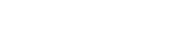Drop a Query

# How to use Numpy Random Function in Python

## How to use numpy.random.rand() function ?

numpy.random.rand() function is used to generate random float values from an uniform distribution over [0,1). These values can be extracted as a single value or in arrays of any dimension.

## Structural overview of numpy.random.rand()

• Syntax: numpy.random.rand(d0, d1, …, dn)
• Purpose: Create an array of the given shape and populate it with random samples from a uniform distribution over [0, 1).
• Parameters:d0, d1, …, dn : int, optional The dimensions of the returned array, must be non-negative. If no argument is given a single Python float is returned.
• Returns out ndarray, shape (d0, d1, …, dn) Random values.
# Import numpy library
import numpy as np

## Implementation of numpy.random.rand() function

numpy.random.rand() function is used to generate random values in the range of [0,1). The data points form an uniform distribution.

Let’s understand it using an example

Step 1: Create a numpy random.rand() function object

randNum = np.random.rand()


Step 2: Call the random.rand() function object

randNum

0.35071131536970257


On calling the random.rand() function, a random float value is returned. This value will always be in the range of [0,1). Also, the value changes on every object call. That is, each time when randNum is called new random value gets generated.

What if you wish to get the same value every time?

## Using Seed to make values static

The values returned by np.random.rand() changes on every consecutive call. By using the np.random.seed() each time when the function is called the same value gets generated.

Let’s see it with an example.

Step 1: Set the seed and create a numpy random.rand() function object

np.random.seed(404)
randNum = np.random.rand()


Step 2: Call the random.rand() function object

randNum

0.6688250856311798


Now, every time the random.rand() function is called, the resultant value would always remain the same.

Till now, we have generated only a single random value. But what if you wish to generate an array of random values?

#### Free Time Series Project Template

Do you want learn how to approach projects across different domains with Time Series?

Get started with your first Time Series Industry Project and Learn how to use and implement algorithms like ARIMA, SARIMA, SARIMAX, Simple Exponential Smoothing and Holt-Winters.#### Do you want learn how to approach projects across different domains with Time Series?

Get started with your first Time Series Industry Project and Learn how to use and implement algorithms like ARIMA, SARIMA, SARIMAX, Simple Exponential Smoothing and Holt-Winters.

## Creating numpy random arrays using rand function

The np.random.rand() function returns a single random float value in the default case. But this function also supports dimensions/shape as input and what this means is that if the shape of the array is passed to the np.random.rand() function, an array containing random values will be returned.

Let us see this with the help of some examples,

### 1-D Arrays by numpy random function

To create one-dimensional random arrays using the np.random.rand() function, pass the shape of the array into rand() function. In this case, the shape of the array is the same as the size of the array.

Step 1: Create a numpy random.rand() function object with shape 10

a_1D_array = np.random.rand(10)


Step 2: Call the random.rand() function object

a_1D_array

array([0.34671278, 0.35893712, 0.72728524, 0.6622387 , 0.60089987,
0.72429985, 0.69959325, 0.01746982, 0.69838873, 0.2936516 ])


On calling the a_1D_array object, an array containing 10 random float values is returned. The returned array is a numpy data type array.

### 2-D Arrays by numpy random function

To create two-dimensional random arrays using the np.random.rand() function, pass the shape of the array in the rand() function. The shape can be passed as (no_of_rows, no_of_columns).

Step 1: Create a numpy random.rand() function object with shape (5,2)

a_2D_array = np.random.rand(5,2)


Step 2: Call the random.rand() function object

a_2D_array

array([[0.98466378, 0.8672886 ],
[0.74133785, 0.35450866],
[0.2958581 , 0.02537018],
[0.1601208 , 0.81913225],
[0.1515311 , 0.72590137]])


On calling the a_2D_array object, an array containing 10 random values of dimension (5,2) is returned.

### 3-D Arrays by numpy random function

To create three-dimensional random arrays using the np.random.rand() function, pass the shape of the array in the rand() function. The shape should be (x-values, y-values, z-values).

Step 1: Create a numpy random.rand() function object with shape (5,2,2)

a_3D_array = np.random.rand(5,2,2)


Step 2: Call the random.rand() function object

a_3D_array

array([[[0.00982155, 0.70143236],
[0.22931261, 0.98901718]],

[[0.58154452, 0.75553604],
[0.03819143, 0.24365719]],

[[0.12186642, 0.52535204],
[0.97041149, 0.0633353 ]],

[[0.35950761, 0.2922847 ],
[0.9058014 , 0.95828723]],

[[0.33627233, 0.46659056],
[0.72309022, 0.73980002]]])


On calling the a_3D_array object, an array containing 20 random values of dimension (5,2,2) is returned.

## Practical Tips

• The np.random.rand() function is useful for scenarios where you want to create some fake data.
• You can use seed() to keep your values same, each and every time the code cell is run.

Q1: The np.random.rand() function can return these values: [1, 2, -2, -0.43]. True or False?

Answer: False. The rand() function returns values in the range [0,1).

Q2: How you can set the state of the random numbers?

Answer: np.random.seed() function can be used to set the state of the random values.

Q3: Write a code to generate a 10x10x3 matrix of random numbers in the range [0,1).

Answer: np.random.rand(10, 10, 3)

References

The article was contributed by Kaustubh G.

## Similar Articles

### Logistic Regression – A Complete Tutorial With Examples in RCourse Preview

## Machine Learning A-Z™: Hands-On Python & R In Data Science

### Free Sample Videos:#### Machine Learning A-Z™: Hands-On Python & R In Data Science#### Machine Learning A-Z™: Hands-On Python & R In Data Science#### Machine Learning A-Z™: Hands-On Python & R In Data Science#### Machine Learning A-Z™: Hands-On Python & R In Data Science